# KR20170047443A - Method and apparatus for processing image date - Google Patents

## Info

Publication number
KR20170047443A
KR20170047443A KR1020150147283A KR20150147283A KR20170047443A KR 20170047443 A KR20170047443 A KR 20170047443A KR 1020150147283 A KR1020150147283 A KR 1020150147283A KR 20150147283 A KR20150147283 A KR 20150147283A KR 20170047443 A KR20170047443 A KR 20170047443A
Authority
KR
South Korea
Prior art keywords
gray level
gray
function
image data
Prior art date
Application number
KR1020150147283A
Other languages
Korean (ko)
Inventor
이명우
Original Assignee
삼성디스플레이 주식회사
Priority date (The priority date is an assumption and is not a legal conclusion. Google has not performed a legal analysis and makes no representation as to the accuracy of the date listed.)
Filing date
Publication date
Application filed by 삼성디스플레이 주식회사 filed Critical 삼성디스플레이 주식회사
Priority to KR1020150147283A priority Critical patent/KR20170047443A/en
Publication of KR20170047443A publication Critical patent/KR20170047443A/en

• 230000000875 corresponding Effects 0 claims description 45
• 238000004364 calculation methods Methods 0 description 5
• 238000000034 methods Methods 0 description 5

## Images

•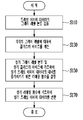•••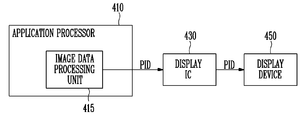•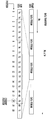•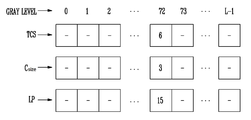•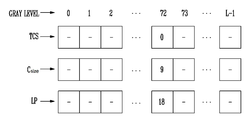•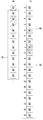•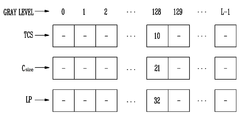•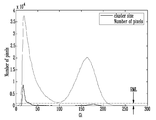•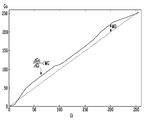•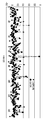•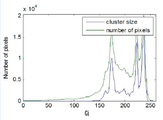•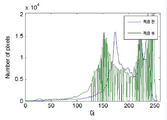•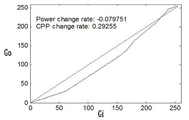•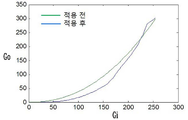## Classifications

• GPHYSICS
• G09EDUCATION; CRYPTOGRAPHY; DISPLAY; ADVERTISING; SEALS
• G09GARRANGEMENTS OR CIRCUITS FOR CONTROL OF INDICATING DEVICES USING STATIC MEANS TO PRESENT VARIABLE INFORMATION
• G09G3/00Control arrangements or circuits, of interest only in connection with visual indicators other than cathode-ray tubes
• G09G3/20Control arrangements or circuits, of interest only in connection with visual indicators other than cathode-ray tubes for presentation of an assembly of a number of characters, e.g. a page, by composing the assembly by combination of individual elements arranged in a matrix no fixed position being assigned to or needed to be assigned to the individual characters or partial characters
• G09G3/2007Display of intermediate tones
• HELECTRICITY
• H04ELECTRIC COMMUNICATION TECHNIQUE
• H04NPICTORIAL COMMUNICATION, e.g. TELEVISION
• H04N5/00Details of television systems
• GPHYSICS
• G09EDUCATION; CRYPTOGRAPHY; DISPLAY; ADVERTISING; SEALS
• G09GARRANGEMENTS OR CIRCUITS FOR CONTROL OF INDICATING DEVICES USING STATIC MEANS TO PRESENT VARIABLE INFORMATION
• G09G3/00Control arrangements or circuits, of interest only in connection with visual indicators other than cathode-ray tubes
• G09G3/20Control arrangements or circuits, of interest only in connection with visual indicators other than cathode-ray tubes for presentation of an assembly of a number of characters, e.g. a page, by composing the assembly by combination of individual elements arranged in a matrix no fixed position being assigned to or needed to be assigned to the individual characters or partial characters
• G09G3/22Control arrangements or circuits, of interest only in connection with visual indicators other than cathode-ray tubes for presentation of an assembly of a number of characters, e.g. a page, by composing the assembly by combination of individual elements arranged in a matrix no fixed position being assigned to or needed to be assigned to the individual characters or partial characters using controlled light sources
• G09G3/30Control arrangements or circuits, of interest only in connection with visual indicators other than cathode-ray tubes for presentation of an assembly of a number of characters, e.g. a page, by composing the assembly by combination of individual elements arranged in a matrix no fixed position being assigned to or needed to be assigned to the individual characters or partial characters using controlled light sources using electroluminescent panels
• G09G3/32Control arrangements or circuits, of interest only in connection with visual indicators other than cathode-ray tubes for presentation of an assembly of a number of characters, e.g. a page, by composing the assembly by combination of individual elements arranged in a matrix no fixed position being assigned to or needed to be assigned to the individual characters or partial characters using controlled light sources using electroluminescent panels semiconductive, e.g. using light-emitting diodes [LED]
• G09G3/3208Control arrangements or circuits, of interest only in connection with visual indicators other than cathode-ray tubes for presentation of an assembly of a number of characters, e.g. a page, by composing the assembly by combination of individual elements arranged in a matrix no fixed position being assigned to or needed to be assigned to the individual characters or partial characters using controlled light sources using electroluminescent panels semiconductive, e.g. using light-emitting diodes [LED] organic, e.g. using organic light-emitting diodes [OLED]
• HELECTRICITY
• H04ELECTRIC COMMUNICATION TECHNIQUE
• H04NPICTORIAL COMMUNICATION, e.g. TELEVISION
• H04N1/00Scanning, transmission or reproduction of documents or the like, e.g. facsimile transmission; Details thereof
• H04N1/46Colour picture communication systems
• H04N1/56Processing of colour picture signals
• H04N1/60Colour correction or control
• H04N1/6027Correction or control of colour gradation or colour contrast
• HELECTRICITY
• H04ELECTRIC COMMUNICATION TECHNIQUE
• H04NPICTORIAL COMMUNICATION, e.g. TELEVISION
• H04N5/00Details of television systems
• H04N5/57Control of contrast or brightness
• HELECTRICITY
• H04ELECTRIC COMMUNICATION TECHNIQUE
• H04NPICTORIAL COMMUNICATION, e.g. TELEVISION
• H04N9/00Details of colour television systems
• H04N9/64Circuits for processing colour signals
• GPHYSICS
• G09EDUCATION; CRYPTOGRAPHY; DISPLAY; ADVERTISING; SEALS
• G09GARRANGEMENTS OR CIRCUITS FOR CONTROL OF INDICATING DEVICES USING STATIC MEANS TO PRESENT VARIABLE INFORMATION
• G09G2320/00Control of display operating conditions
• G09G2320/02Improving the quality of display appearance
• G09G2320/0271Adjustment of the gradation levels within the range of the gradation scale, e.g. by redistribution or clipping
• GPHYSICS
• G09EDUCATION; CRYPTOGRAPHY; DISPLAY; ADVERTISING; SEALS
• G09GARRANGEMENTS OR CIRCUITS FOR CONTROL OF INDICATING DEVICES USING STATIC MEANS TO PRESENT VARIABLE INFORMATION
• G09G2320/00Control of display operating conditions
• G09G2320/02Improving the quality of display appearance
• G09G2320/029Improving the quality of display appearance by monitoring one or more pixels in the display panel, e.g. by monitoring a fixed reference pixel
• GPHYSICS
• G09EDUCATION; CRYPTOGRAPHY; DISPLAY; ADVERTISING; SEALS
• G09GARRANGEMENTS OR CIRCUITS FOR CONTROL OF INDICATING DEVICES USING STATIC MEANS TO PRESENT VARIABLE INFORMATION
• G09G2320/00Control of display operating conditions
• G09G2320/066Adjustment of display parameters for control of contrast
• GPHYSICS
• G09EDUCATION; CRYPTOGRAPHY; DISPLAY; ADVERTISING; SEALS
• G09GARRANGEMENTS OR CIRCUITS FOR CONTROL OF INDICATING DEVICES USING STATIC MEANS TO PRESENT VARIABLE INFORMATION
• G09G2330/00Aspects of power supply; Aspects of display protection and defect management
• G09G2330/02Details of power systems and of start or stop of display operation
• G09G2330/021Power management, e.g. power saving
• GPHYSICS
• G09EDUCATION; CRYPTOGRAPHY; DISPLAY; ADVERTISING; SEALS
• G09GARRANGEMENTS OR CIRCUITS FOR CONTROL OF INDICATING DEVICES USING STATIC MEANS TO PRESENT VARIABLE INFORMATION
• G09G2360/00Aspects of the architecture of display systems
• G09G2360/16Calculation or use of calculated indices related to luminance levels in display data

## Abstract

A method of processing image data according to an embodiment of the present invention includes a step of detecting the gray level distribution of frame image data, a step of calculating a cluster size for each gray level based on the detected gray level distribution, a step of determining a remapping function to increase the contrast of the frame image data based on the gray level distribution and the cluster size, and a step of converting the frame image data based on the remapping function.

## Description

TECHNICAL FIELD  The present invention relates to a method and an apparatus for processing image data,

The present invention relates to a method and an apparatus for processing image data.

2. Description of the Related Art In recent years, a cathode ray tube (CRT) has been replaced by a liquid crystal display (LCD) in accordance with such a demand. However, a liquid crystal display device requires a separate backlight as a light-emitting device, and has many problems in terms of response speed and viewing angle.

Recently, organic light emitting diode (OLED) displays have been attracting attention as display devices capable of overcoming such problems. The organic light emitting diode display includes two electrodes and a light emitting layer disposed therebetween. Electrons injected from one electrode and holes injected from the other electrode are combined in the light emitting layer to form an exciton And the exciton emits light while emitting energy.

Since the organic light emitting display device is of a self-emission type and requires no separate light source, it is advantageous not only in power consumption but also in response speed, viewing angle, and contrast ratio. Here, the light emitting layer is made of an organic material that uniquely emits any one of primary colors such as red, green, and blue primary colors, and displays a desired image by a spatial sum of basic color light emitted by the light emitting layer. On the other hand, a method of processing image data has been a major concern in order to improve the visibility of displayed images.

One embodiment of the present invention provides a method of processing image data that can improve the contrast of the displayed image.

An embodiment of the present invention provides an image data processing apparatus capable of improving contrast of an image to be displayed.

A method of processing image data according to an embodiment of the present invention includes the steps of detecting a gray level distribution of frame image data, computing a cluster size for each gray level based on the detected gray level distribution, Determining a remapping function to increase the contrast of the frame image data based on the gray level distribution and the cluster size, and converting the frame image data based on the remapping function .

In one embodiment, in the step of detecting the gray level distribution of the frame image data, the number of pixel data belonging to each gray level among the pixel data belonging to the frame image data may be counted.

In one embodiment, in calculating the cluster size for each gray level, it is possible to calculate the degree to which the pixel data corresponding to the gray levels are located closely in the frame.

In one embodiment, the remapping function may be determined by the following equation.

G (g) = G (g-1) + d (g)

G (g) is the remapped gray level corresponding to the gray level g, d (g) is the gray level distribution and depends on the cluster size. In the above equation, g is the gray level of each, G .

In one embodiment, the d (g) function may be determined by the following equation.

d (g) a = 1 / MAX grad, R ( g-1) = 1, | if the <MAX gray _ diff | G ( g) -g

In the equation, MAX grad is a value that is determined in advance, and the maximum rate of change of the remapping function, gray MAX _ diff is a value that is determined in advance, and the maximum difference value with the remapping function and the original function of the map. R () is a function of the low distribution level of the gray level and R (g) is the low distribution of the gray level g.

In one embodiment, the R (g) function may be determined by the following equation.

If R (g) = 1, H (g) < RML, and CSize (g) = 0,

R (g) = 0, H (g)? RML, or CSize (g)? 0

Where G (g) is the number of pixel data corresponding to the gray level g, Csize (g) is the cluster size corresponding to the gray level g, RML is the predetermined value, gray scales are the other gray levels Value &lt; / RTI &gt;

In one embodiment, computing the cluster size for each of the gray levels comprises: determining clusters in which two or more pixels corresponding to a gray level g for each of the rows in the frame are formed within a predetermined proximity value, And determine the cluster size Csize (g) based on the number of pixels included in all the clusters in the frame.

In one embodiment, it may be determined that the two pixels are included in the same cluster when the distance between two pixels corresponding to the gray level g is less than or equal to a reference distance.

In one embodiment, computing the cluster size for each of the gray levels comprises: determining clusters in which two or more pixels corresponding to a gray level g for each of the rows in the frame are formed within a predetermined proximity value, And determine the cluster size Csize (g) based on the number of pixels included in the clusters having a certain size or larger among the clusters.

In one embodiment, when R (g-1) = 0, the remapping function may be determined by the following equation.

G (g) = g

In one embodiment, the d (g) function may be determined by the following equation.

In the above equation, Grad (g) is a function depending on the degree of low distribution of gray level values greater than g, and MAX grad is a predetermined value, which is the maximum rate of change of the remapping function.

In one embodiment, the Grad (g) function may be determined by the following equation.

Csize (g) is the cluster size corresponding to the gray level g, TCsize is the sum of the cluster sizes of all gray levels, and R (g) is the low distribution of the gray level g.

In one embodiment, the R (g) function may be determined by the following equation.

If R (g) = 1, H (g) < RML, and CSize (g) = 0,

R (g) = 0, H (g)? RML, or CSize (g)? 0

Where G (g) is the number of pixel data corresponding to the gray level g, Csize (g) is the cluster size corresponding to the gray level g, RML is the predetermined value, gray scales are the other gray levels Value &lt; / RTI &gt;

In one embodiment, the Grad (g) function can be determined by the following equation

In the above equation, Csize (g) is the cluster size corresponding to the gray level g, and TCsize is the sum of the cluster sizes of all gray levels.

An image data processing apparatus according to an embodiment of the present invention includes a cluster calculation unit, a gray remapping unit, and a filter unit. The cluster calculator detects the distribution of the gray levels of the frame image data and calculates the cluster size for each gray level. The gray remapping section determines a remapping function to increase the contrast of the frame image data based on the distribution of the gray levels and the cluster size. The filter unit converts the frame image data based on the remapping function.

In one embodiment, the cluster calculator may detect a distribution of the gray levels by counting the number of pixel data belonging to each gray level among pixel data belonging to the frame image data.

In one embodiment, the cluster calculator may calculate the cluster size by calculating the degree of proximity of the pixel data corresponding to the gray levels in the frame.

In one embodiment, the gray remapping unit may determine the remapping function by the following equation.

If the <MAX gray _ diff, | G (g) = G (g-1) + 1 / MAX grad, and R (g-1) = 1 , | G (g) -g

When G (g) = g and R (g-1) = 0

In the equation, MAX grad is a value that is determined in advance, and the maximum rate of change of the remapping function, gray MAX _ diff is a value that is determined in advance, and the maximum difference value with the remapping function and the original function of the map. R () is a function of the low distribution level of the gray level and R (g) is the low distribution of the gray level g.

In one embodiment, the gray remapping portion may determine the R (g) function by the following equation.

If R (g) = 1, H (g) < RML, and CSize (g) = 0,

R (g) = 0, H (g)? RML, or CSize (g)? 0

Where G (g) is the number of pixel data corresponding to the gray level g, Csize (g) is the cluster size corresponding to the gray level g, RML is the predetermined value, gray scales are the other gray levels Value &lt; / RTI &gt;

In one embodiment, the gray remapping unit may determine the remapping function by the following equation.

When the 1, - G (g) = G (g-1) + MAX grad - 1, Grad (g) ≥ MAX grad

In the above equation, Grad (g) is a function depending on the degree of low distribution of gray level values greater than g, and MAX grad is a predetermined value, which is the maximum rate of change of the remapping function.

According to an embodiment of the present invention, it is possible to provide a method of processing image data capable of improving contrast of an image to be displayed.

According to an embodiment of the present invention, it is possible to provide an image data processing apparatus capable of improving the contrast of a displayed image.

1 is a flowchart showing a method of processing image data according to an embodiment of the present invention.
2 is a block diagram illustrating an imaging system including an image data processing apparatus in accordance with an embodiment of the present invention.
3 is a block diagram showing an exemplary embodiment of the image data processing unit shown in Fig.
4 is a block diagram illustrating an imaging system including an apparatus for processing image data according to another embodiment of the present invention.
5A, 5B and 5C are diagrams for explaining a method of calculating a cluster size for processing image data according to an embodiment of the present invention.
6A and 6B are diagrams for explaining another method of calculating a cluster size for processing image data according to an embodiment of the present invention.
7 is a graph illustrating a result of calculating a cluster size for processing image data according to an embodiment of the present invention.
8 is a graph illustrating an example of a remapping function calculated to process image data in accordance with an embodiment of the present invention.
9 is a graph showing a result of performing a method of processing image data according to an embodiment of the present invention on 148 standard images.
10A, 10B, 10C, and 10D are graphs showing results of performing image data processing methods according to an exemplary embodiment of the present invention on an exemplary image.

Hereinafter, preferred embodiments of the present invention will be described in detail with reference to the accompanying drawings. Note that, in the drawings, the same components are denoted by the same reference symbols as possible. In the following description, only parts necessary for understanding the operation according to the present invention will be described, and descriptions of other parts will be omitted in order to avoid obscuring the gist of the present invention. Further, the present invention is not limited to the embodiments described herein but may be embodied in other forms. It is to be understood, however, that the invention may be embodied in many other specific forms without departing from the spirit or essential characteristics thereof.

1 is a flowchart showing a method of processing image data according to an embodiment of the present invention.

Referring to FIG. 1, a method of processing image data according to an exemplary embodiment of the present invention includes detecting (S 110) a gray level distribution of frame image data, (S150) of determining a remapping function for increasing the contrast of the frame image data based on the gray-level distribution and the cluster size, (S170) converting the frame image data based on the frame image data.

In the step of detecting the gray level distribution of the frame image data (S110), it is possible to analyze input frame image data and calculate how many pixels in the image data have a gray level corresponding to the corresponding gray level. The gray level (g) is 0, 1, 2, ... , And L-1. For example, when the gray level (g) of the frame image data is represented by 8 bits, the total number L of gray levels is 256 and the gray level (g) has integer values from 0 to 255. In step S110 of detecting the gray level distribution of the frame image data, the number of pixels corresponding to each of the gray levels g may be calculated as a distribution of gray levels H (g). The H (g) is 0, 1, 2, ... , And the gray level (g) of L-1.

In step S130 of calculating the cluster size for each gray level based on the detected gray level distribution, the gray level distribution (H (g)) is calculated based on the gray level distribution H (g) calculated in step S110 g) representing a degree of proximity that indicates the degree to which pixels corresponding to each of the pixels G, G are positioned close together in the frame. The method of calculating the cluster size based on the distribution of the gray levels will be described later with reference to Figs. 5A, 5B, 5C, 6A and 6B.

In step S150 of determining a remapping function for increasing the contrast of the frame image data based on the gray level distribution and the cluster size, the gray level distribution H (g (G)) based on the cluster size Csize (g) calculated in step S130) and the cluster size Csize (g) calculated in step S130. A specific method of determining the remapping function G (g) based on the gray level distribution H (g) and the cluster size Csize (g) will be described later with reference to Figs. 7 and 8. Fig.

In the step of transforming the frame image data based on the remapping function (S170), the image data may be transformed by applying the remapping function G (g) determined in the step 150 to the inputted frame image data.

2 is a block diagram illustrating an imaging system including an image data processing apparatus in accordance with an embodiment of the present invention.

Referring to FIG. 2, the image system includes a display IC 200 and a display device 250. In addition, the display IC 200 includes a frame memory 210 and an image data processing section 230.

The frame memory 210 may buffer the input frame image data (ID) and provide the buffered image data to the image data processing unit 230. In an exemplary image system, image data in the RGB format can be converted into YCbCr format data by applying a transform function. The YCbCr format is represented by a luminance value Y and chrominance values Cb and Cr. Since the human eye is more sensitive to brightness than the color, the YCbCr format may be effective. For example, the brightness value Y may represent a gray level (g).

The image data processing unit 230 detects the gray level distribution H (g) by analyzing the received frame image data ID and calculates the cluster size Csize (g) to obtain the remapping function G (g) , And convert the inputted frame image data (ID) based on the determined remapping function G (g). More specifically, the image data processing unit 230 may perform a remapping operation to increase the contrast of the frame image data (ID) based on the distribution of the gray levels H (g) and the cluster size Csize (g) The function G (g) can be determined. In addition, the image data processing unit 230 may apply the determined remapping function G (g) to the frame image data (ID) to generate the converted frame image data (PID). The converted frame image data (PID) is image data in which the gray level is remapped so that the contrast of the frame image data (ID) is increased. In this case, the image data processing unit 230 shown in FIG. 2 can operate as an image data processing apparatus according to an embodiment of the present invention. The specific configuration of the image data processing unit 230 will be described later with reference to FIG.

Display device 250 may display the converted frame image data (PID) output from the display (IC). The converted frame image data (PID) is image data in which the gray level is remapped so that the contrast of the frame image data (ID) is increased. Therefore, the image displayed on the display device 250 is an enhanced contrast image. Therefore, the visibility of the displayed image can be improved.

3 is a block diagram showing an exemplary embodiment of the image data processing unit shown in Fig.

Referring to FIG. 3, the image data processing unit 300 includes a cluster calculation unit 310, a gray remapping unit 330, and a filter unit 350.

The cluster calculator 310 detects the distribution H (g) of the gray levels g of the frame image data ID and calculates the cluster size Csize (g) for each gray level. The cluster calculator 310 may also calculate a function R (g) representing the degree of low distribution of the gray level g based on the distribution H (g) of the detected gray levels.

The gray remapping unit 330 may use the remapping function G (g (g)) to increase the contrast of the frame image data based on the distribution of gray levels H (g) and the cluster size Csize ) Can be determined.

The function R (g) indicating the degree of low distribution of the gray level g may be calculated by the cluster calculating unit 310 and transmitted to the gray remapping unit 330 as shown in FIG. 3, (330) itself. In this case, the cluster calculator 310 can also deliver the distribution (H (g)) of gray levels in addition to the cluster size (Csize (g)) to the gray remapping unit 330. The gray remapping section 330 is a function that represents a low distribution degree of the gray level g based on the cluster size Csize (g) and the distribution H (g) of the gray levels g )) Can be calculated.

The function R (g) indicating the degree of low distribution of the gray level g is a function having a value of 0 or 1 for each gray level value. Gray level remapping is required in calculating the remapping function (G (g)) for improving the contrast. In this case, the function R (g) indicates whether the corresponding gray level can be integrated with another gray level. When the value of R (g) is 0, it indicates that the corresponding gray level (g) can not be integrated with another gray level. A value of R (g) of 1 indicates that the corresponding gray level (g) can be integrated with another gray level. For example, if R (84) = 1 pixel data having a gray level of 85 can be remapped to a gray level of 84, if pixel data having a gray level of 85 is to be remapped to a lower gray level. However, when R (84) = 0, pixel data having a gray level of 85 can not be remapped to a gray level of 84. [

If there is a large amount of pixel data corresponding to a certain gray level, that is, H (g) has a large value, the overall contrast may be lowered despite gray level remapping if the gray level is integrated with another gray level. Therefore, only in the case of a gray level having a low value of H (g), it is possible to set the value of R (g) to 1 so as to be able to integrate with other gray level values, and a gray level The value of R (g) may be set to 0 so as not to be integrated with other gray levels.

On the other hand, when the cluster exists in the corresponding gray level g and CSize (g)? 0 is associated with the cluster size Csize (g) to be described later, Is likely to be included. In this case, when the gray level is integrated with other gray levels, the visibility of the shape may be degraded. Therefore, the value of R (g) may be set to 0 even when CSize (g) ≠ 0.

The setting of the function R (g) value will be described later with reference to Figs. 5A, 5B, 5C, 6A and 6B together with a method of calculating the cluster size Csize (g).

The filter unit 350 may convert the frame image data ID into the converted frame image data PID based on the remapping function G (g).

Thus, by processing the frame image data (ID) based on the distribution of gray levels H (g), cluster size Csize (g), and function R (g) The visibility can be improved.

4 is a block diagram illustrating an imaging system including an apparatus for processing image data according to another embodiment of the present invention.

4, the imaging system includes an application processor 410, a display IC 430, and a display device 450. On the other hand, the application processor 410 includes an image data processing unit 415. In this case, the image data processing unit 415 shown in FIG. 4 can operate as an image data processing apparatus according to an embodiment of the present invention.

Unlike the imaging system of FIG. 2, the image data processing unit 415 in the imaging system of FIG. 4 is included in the application processor 410 rather than the display IC 430. In this case, the frame image data generated by the application processor 410 is converted into the converted frame image data (PID) in the image data processing unit 415 in the application processor 410 and transferred to the display IC 430. Meanwhile, the display IC 430 transmits the received conversion frame image data (PID) to the display device 450. The display device 450 displays the received converted frame image data (PID).

As shown in FIGS. 2 and 4, the image data processing units 230 and 415 may be included in the display IC, but may be included in the application processor. That is, a method of processing image data according to an embodiment of the present invention may be performed by a display IC, or may be performed by an application processor. In this case, those skilled in the art will appreciate that at least some of the components of the image data processing unit may be implemented in the form of a product, etc., including computer-readable program code stored in a computer-readable medium. The computer readable program code may be provided to a processor of an application processor or other data processing apparatus.

5A, 5B and 5C are diagrams for explaining a method of calculating a cluster size for processing image data according to an embodiment of the present invention.

Referring to FIG. 5A, frame image data may be provided in the form of a stream from an external device such as an application processor (AP), an image signal processor (ISP), and the like. As shown in FIG. 5A, one frame data includes Nv row data, and one row data may include Nh pixel data. In FIG. 3, only gray levels are shown among the pixel data for convenience.

When counting the number of gray levels of pixel data sequentially input in stream form, the distribution (H (g)) of gray levels is obtained as a histogram. That is, the distribution H (g) of the gray levels corresponds to a histogram representing the number of pixels corresponding to each of the gray levels g.

Referring to Figures 5A, 5B and 5C, a method of calculating the cluster size representing the proximity to each of the gray levels will be described.

The locality represents the degree to which the pixels corresponding to each of the gray levels are located close together within the frame. That is, the degree of proximity indicates the degree to which the same gray levels form a cluster, that is, a cluster, close to each other. Since the image data is input in each row, the calculation of the locality can be performed on a row-by-row basis. Three vectors may be used for the calculation of the proximity. A temporary cluster size vector (TCS) for storing the number of pixels of each cluster, a cluster size vector (Csize) representing a total number of pixels of the cluster in one frame, And a last position vector (LP) that stores the last indicated position.

If the value obtained by subtracting the value of the last position vector LP of the corresponding gray level from the current position is less than a predetermined value, the value of the temporary cluster size vector TCS of the gray level is set to 1 . On the other hand, if the value obtained by subtracting the value of the last position vector LP of the corresponding gray level from the current position is greater than the predetermined value, the value of the temporary cluster size vector TCS is added to the cluster size vector Csize, (TCS) is initialized to zero. And updates the value of the final position vector LP to the current position.

In this way, the value of the cluster size vector Csize of each gray level can be calculated by calculating for all the rows of one frame image data. By integrating these cluster size vectors, a function for the gray level (g) Can be generated with a cluster size (Csize (g)). This cluster size (Csize (g)) represents the proximity of each gray level (g).

Thus, for each of the rows in the frame, detect clusters in which two or more pixels corresponding to g are formed in close proximity to each other, and calculate a cluster size Csize (g) based on the number of pixels included in all clusters in the frame. ) Can be set. It can be determined that the two pixels are included in the same cluster when the distance between the two pixels corresponding to the g is less than or equal to the reference distance.

FIG. 5B shows vector values before reflecting the current gray level, and FIG. 5C shows vector values after reflecting the current gray level. In the example of FIGS. 5B and 5C, if the current position at which the data is read is position 18, the current gray level is 72 and the corresponding final position vector value is 15. If the reference distance is 2, the difference between the current position and the final position is 3, which is greater than 2. Therefore, 6, which is the value of the temporary cluster size vector TCS, is added to 3, which is the value of the cluster size vector Csize, ) Is 9. On the other hand, the value of the temporary cluster size vector TCS is initialized to 0, and the value of the last position vector LP is updated to 18, which is the current position.

6A and 6B are diagrams for explaining another method of calculating a cluster size for processing image data according to an embodiment of the present invention.

Referring to FIG. 6A, gray level values for 20 pixels are shown on row A, and gray level values for up to 13 pixels are shown on row B. FIG. In another method of calculating the cluster size according to an embodiment of the present invention, a difference between a gray level value formed in a vicinity of a predetermined proximity distance value in an input data stream and a gray level of a current position is set to a predetermined threshold value GDth), the value of the temporary cluster size vector TCS of the current gray level value is increased. As shown in FIG. 6A, the fifth data in the row B has 128 gray level values, and the last position in which 128 gray level values appear is the second position in the row B. The distance between the current position and the last position in which the 128 gray level value appears is 3, and the predetermined proximity value is 2, so if the method described with reference to Figs. 5A to 5C is followed, The gray level value of 128 does not form a cluster and will not increase the value of the cluster size vector TCS. However, according to the method described with reference to Figs. 6A and 6B, there are 129 gray level values between the second position of the row B and the gray level values of 128 located at the fifth row. When the predetermined threshold value GDth is set to 2, since the difference between the gray level values of 128 and 129 is smaller than the threshold value GDth as 1, the temporary 128 gray level value at the position of the 5th pixel data of the row B The cluster size vector (TCS) increases by one.

That is, according to the method according to Figs. 5A to 5C, the temporary cluster size vector (TCS) increases by 9 for the 128 gray levels shown in the B row. And the 128 gray level value of the B row second position does not form a cluster with the 128 gray levels thereafter. However, according to the method described with reference to Figs. 6A and 6B, the 128 gray levels included in the pixel data D3 form a cluster. Thus, for a 128 gray level shown in row B, the temporary cluster size vector (TCS) increases by 10.

According to the method of determining the cluster size C (g) according to an embodiment of the present invention, the value is discarded for a temporary cluster size vector TCS less than a certain size (hereinafter referred to as OBJsize). That is, the cluster size vector Csize is added only to the temporary cluster size vector TCS equal to or larger than the predetermined size OBJsize. Referring to FIG. 6A, the temporary cluster size vector TCS for the pixel data D1 in the row A is 5, the temporary cluster size vector TCS for the pixel data D2 in the row A is 11, The temporary cluster size vector TCS for pixel data D3 in row B is 10. Therefore, when the predetermined size OBJsize is 8, the temporary cluster size vector TCS for the pixel data D1 is discarded and the temporary cluster size vector TCS for the pixel data D2, Is added to the cluster size vector Csize.

7 is a graph illustrating a result of calculating a cluster size for processing image data according to an embodiment of the present invention.

Referring to Fig. 7, a distribution (H (g)) and a cluster size (Csize (g)) of gray levels included in the frame image data are shown. Also shown is a comparison value RML for determining R (g). The distribution of gray levels (H (g)) is shown in Number of pixels and the cluster size (Csize (g)) is shown in cluster size. On the other hand, the comparison value RML for determining R (g) is 1500.

In such a case, R (g) of the gray level (g) is determined to be 1 only when H (g) <1500 and CSize (g) = 0. Otherwise, R (g) is determined to be 0 if H (g) ≥ RML, or if CSize (g) ≠ 0.

In the method of processing image data according to an embodiment of the present invention, the remapping function G (g) can be determined by the following equation (1).

G (g) = G (g-1) + d (g)

Where d (g) is a function that depends on the gray level distribution and the cluster size.

First, it is determined whether or not the value of the remapping function G (g) can be remapped to a value smaller than the original value g. R (g-1) = 1 and, | G (g) -g | <MAX gray _ to a, d (g) functions only when the diff may be determined by equation (2).

d (g) = 1 / MAX grad --- (2)

In Equation 2, MAX grad is a value that is determined in advance, and the maximum rate of change of the remapping function, gray MAX _ diff is a value that is determined in advance, and the maximum difference value with the remapping function and the original function of the map. R () is a function of the low distribution level of the gray level and R (g) is the low distribution of the gray level g.

Further, the R (g) function can be determined by the following equations (3) and (4).

(3) when R (g) = 1, H (g) < RML and CSize

R (g) = 0, H (g)? RML, or CSize (g)

The RML is a predetermined value and may be a threshold of the number of pixel data for determining whether the gray-scale can be merged with other gray-level values in the remapping step. In the example described above with reference to FIG. 7, the RML value may be predetermined to 1500.

If the value of the remapping function G (g) can not be remapped to a value smaller than the original value g, i.e., R (g-1) = 0, It can be remapped to a value larger than the original value (g).

That is, when R (g-1) = 0, the d (g) function can be determined by the following equations (5) and (6).

In the above equations (5) and (6), Grad (g) is a function depending on the degree of low distribution of gray level values larger than g, and MAX grad is a predetermined value, which is the maximum rate of change of the remapping function.

In one embodiment, the Grad (g) function may be determined by Equation (7) below.

--- (7)

In Equation (7), TCsize is the sum of the cluster sizes of all gray levels.

In another embodiment, the Grad (g) function may be determined by the following equation (8).

--- (8)

With the above expression (8), the power consumption in the organic light emitting display device can be lowered. That is, the contrast can be slightly increased while lowering the power consumption.

In another embodiment, when the value of the remapping function G (g) can not be remapped to a value less than the original value g, i.e., when R (g-1) = 0, the remapping function G g) can be mapped to the original value (g). In this case, since the calculation of the remapping function G (g) is simple, the power consumption can be reduced.

The above process can be sequentially performed from g having the smallest gray scale value to g having the highest gray scale value. For example, if the gray scale value is defined as a value between 0 and 255, the value of G (g) is obtained starting from a g value of 0 and sequentially reaching a value of 255 g. For each g value, determine whether G (g) can be remapped to a value lower than the current g, and if possible, G (g) is determined to be a value lower than the current g. If G (g) can not be remapped to a value lower than the current g, it is judged whether or not remapping to a value higher than the present g is possible. If possible, G (g) is determined to be a value higher than the current g. Therefore, the contrast of the image data can be improved.

8 is a graph illustrating an example of a remapping function calculated to process image data in accordance with an embodiment of the present invention.

The remapping function G (g) calculated according to the above-described method is shown in Fig. In FIG. 8, MD may be the maximum difference value between the remapping function and the original mapping function. MD That may be the value MAX gray _ diff. Also, the maximum value of? Go /? Gi, that is, the slope of the remapping function is smaller than MG. That is, MG is the maximum rate of change of the remapping function and may be the same value as the above-mentioned MAX grad .

That is, the remapping function of the original mapping function and the MAX gray _ more than diff without chayinaji, it may be determined that changes in the rate of change within the MAX grad.

9 is a graph showing a result of performing a method of processing image data according to an embodiment of the present invention on 148 standard images.

Referring to FIG. 9, when an image is processed according to the method of processing image data according to an embodiment of the present invention, the contrast per pixel (CPP) value is increased as a whole. In addition, since the gray level value forming the shape is mainly changed, the visibility of the image can be improved.

Table 1 below shows power and CPP changes when an image is processed according to a method of processing image data according to an embodiment of the present invention. The power before processing (Power_1), the power after processing (Power_2), the rate of power change (ΔPower_r), the CPP before processing (CPP_1), the CPP after processing (CPP_2) and the CPP change rate (ΔCPP_r) are shown.

Power_1 Power_2 ΔPower_r CPP_1 CPP_2 ? CPP_r 103.60 109.60 0.11 3.55 3.84 0.10

As a result, the power consumption is slightly increased but the contrast is improved.

10A, 10B, 10C, and 10D are graphs showing results of performing image data processing methods according to an exemplary embodiment of the present invention on an exemplary image.

10A is a graph showing a pixel distribution and a cluster size, FIG. 10B is a graph showing a pixel distribution after conversion, FIG. 10C is a graph showing a remapping function, and FIG. 10D is a graph showing gamma after conversion. That is, the contrast of the image can be improved by the method according to the present invention, thereby improving the visibility.

At this point, it will be appreciated that the combinations of blocks and flowchart illustrations in the process flow diagrams may be performed by computer program instructions. These computer program instructions may be loaded into a processor of a general purpose computer, special purpose computer, or other programmable data processing apparatus, so that those instructions, which are executed through a processor of a computer or other programmable data processing apparatus, Thereby creating means for performing functions. These computer program instructions may be stored in a computer readable memory or in a computer capable of directing a computer or other programmable data processing apparatus to implement a function in a particular manner, It is also possible for instructions stored in memory to produce manufacturing items that contain instruction means for performing the functions described in the flowchart block (s). Computer program instructions may also be stored on a computer or other programmable data processing equipment so that a series of operating steps may be performed on a computer or other programmable data processing equipment to create a computer- It is also possible for the instructions to perform the processing equipment to provide steps for executing the functions described in the flowchart block (s).

In addition, each block may represent a module, segment, or portion of code that includes one or more executable instructions for executing the specified logical function (s). It should also be noted that in some alternative implementations, the functions mentioned in the blocks may occur out of order. For example, two blocks shown in succession may actually be executed substantially concurrently, or the blocks may sometimes be performed in reverse order according to the corresponding function.

Herein, the term &quot; part &quot; used in the present embodiment means a hardware component such as software or an FPGA or an ASIC, and 'part' performs certain roles. However, 'part' is not meant to be limited to software or hardware. &Quot; to &quot; may be configured to reside on an addressable storage medium and may be configured to play one or more processors. Thus, by way of example, 'parts' may refer to components such as software components, object-oriented software components, class components and task components, and processes, functions, , Subroutines, segments of program code, drivers, firmware, microcode, circuitry, data, databases, data structures, tables, arrays, and variables. The functions provided in the components and components may be further combined with a smaller number of components and components or further components and components. In addition, the components and components may be implemented to play back one or more CPUs in a device or a secure multimedia card.

The embodiments of the present invention disclosed in the present specification and drawings are merely illustrative examples of the present invention and are not intended to limit the scope of the present invention in order to facilitate understanding of the present invention. It will be apparent to those skilled in the art that other modifications based on the technical idea of the present invention are possible in addition to the embodiments disclosed herein.

200: display IC 210: frame memory
240: image data processing unit 250: display device

## Claims (20)

1. Detecting a gray level distribution of the frame image data;
Calculating a cluster size for each gray level based on the detected gray level distribution;
Determining a remapping function to increase the contrast of the frame image data based on the gray-level distribution and the cluster size; And
And converting the frame image data based on the remapping function.
2. The method according to claim 1,
In the step of detecting the gray level distribution of the frame image data,
And counting the number of pixel data belonging to each gray level among pixel data belonging to the frame image data.
3. 3. The method of claim 2,
In the step of calculating the cluster size for each gray level,
And calculating the degree of proximity of the pixel data corresponding to the gray levels within the frame.
4. The method according to claim 1,
Wherein the remapping function is determined by: &lt; EMI ID = 17.0 &gt;

G (g) = G (g-1) + d (g)

G (g) is the remapped gray level corresponding to the gray level g, d (g) is the gray level distribution and depends on the cluster size. In the above equation, g is the gray level of each, G .
5. 5. The method of claim 4,
Wherein the d (g) function is determined by the following equation.

d (g) a = 1 / MAX grad, R ( g-1) = 1, | if the <MAX gray _ diff, | G (g) -g

In the equation, MAX grad is a value that is determined in advance, and the maximum rate of change of the remapping function, gray MAX _ diff as the value is to be determined in advance, the remapping function and the maximum difference ¹ of the original mapping function. R () is a function of the low-level distribution of the gray level, and R (g) is the low-level distribution of the gray level g.
6. 6. The method of claim 5,
Wherein the R (g) function is determined by the following equation.

If R (g) = 1, H (g) < RML, and CSize (g) = 0,
If R (g) = 0, H (g) &gt; RML, or CSize (g)

Where G (g) is the number of pixel data corresponding to the gray level g, Csize (g) is the cluster size corresponding to the gray level g, RML is the predetermined value, gray scales are the other gray levels Value &lt; / RTI &gt;
7. The method according to claim 6,
In the step of calculating the cluster size for each gray level,
Detecting clusters in which two or more pixels corresponding to a gray level g for each of the rows in the frame are formed within a predetermined proximity distance value, and detecting cluster sizes based on the number of pixels included in all clusters in the frame Csize &lt; / RTI &gt; (g).
8. 8. The method of claim 7,
And determining that the two pixels are included in the same cluster when the distance between two pixels corresponding to the gray level g is less than or equal to a reference distance.
9. The method according to claim 6,
In the step of calculating the cluster size for each gray level,
Detecting clusters in which two or more pixels corresponding to the gray level g are formed within a predetermined proximity distance value for each of the rows in the frame, based on the number of pixels included in clusters of a certain size or larger among the clusters To determine the cluster size Csize (g).
10. 6. The method of claim 5,
And R (g-1) = 0, the remapping function is determined by the following equation.
G (g) = g.
11. 5. The method of claim 4,
Wherein the d (g) function is determined by the following equation.

In the above equation, Grad (g) is a function depending on the degree of low distribution of gray level values larger than g, and MAX grad is a predetermined value, which is the maximum rate of change of the remapping function.
12. 12. The method of claim 11,
Wherein the Grad (g) function is determined by the following equation.

Csize (g) is the cluster size corresponding to the gray level g, TCsize is the sum of the cluster sizes of all gray levels, and R (g) is the low distribution of the gray level g.
13. 13. The method of claim 12,
Wherein the R (g) function is determined by the following equation.

If R (g) = 1, H (g) < RML, and CSize (g) = 0,
If R (g) = 0, H (g) &gt; RML, or CSize (g)

Where G (g) is the number of pixel data corresponding to the gray level g, Csize (g) is the cluster size corresponding to the gray level g, RML is the predetermined value, gray scales are the other gray levels Value &lt; / RTI &gt;
14. 12. The method of claim 11,
Wherein the Grad (g) function is determined by the following equation.

In the above equation, Csize (g) is the cluster size corresponding to the gray level g, and TCsize is the sum of the cluster size of the entire gray levels.
15. A cluster calculator for detecting a distribution of gray levels of the frame image data and calculating a cluster size for each gray level;
A gray remapping unit for determining a remapping function for increasing the contrast of the frame image data based on the distribution of the gray levels and the cluster size; And
And a filter unit for converting the frame image data based on the remapping function.
16. 16. The method of claim 15,
Wherein the cluster calculator counts the number of pixel data belonging to each gray level among the pixel data belonging to the frame image data and detects the distribution of the gray levels.
17. 17. The method of claim 16,
Wherein the cluster calculator calculates the cluster size by calculating the degree of proximity of the pixel data corresponding to the gray levels in the frame.
18. 16. The method of claim 15,
Wherein the gray remapping unit determines the remapping function by the following equation.

If the <MAX gray _ diff, | G (g) = G (g-1) + 1 / MAX grad, and R (g-1) = 1 , | G (g) -g
If G (g) = g and R (g-1) = 0,

In the equation, MAX grad is a value that is determined in advance, and the maximum rate of change of the remapping function, gray MAX _ diff as the value is to be determined in advance, the remapping function and the maximum difference ¹ of the original mapping function. R () is a function of the low-level distribution of the gray level, and R (g) is the low-level distribution of the gray level g.
19. 19. The method of claim 18,
Wherein the gray remapping unit determines the R (g) function by the following equation.

If R (g) = 1, H (g) < RML, and CSize (g) = 0,
If R (g) = 0, H (g) &gt; RML, or CSize (g)

Where G (g) is the number of pixel data corresponding to the gray level g, Csize (g) is the cluster size corresponding to the gray level g, RML is the predetermined value, gray scales are the other gray levels Value &lt; / RTI &gt;
20. 16. The method of claim 15,
Wherein the gray remapping unit determines the remapping function by the following equation.

When the 1, - G (g) = G (g-1) + MAX grad - 1, Grad (g) ≥ MAX grad

In the above equation, Grad (g) is a function depending on the degree of low distribution of gray level values larger than g, and MAX grad is a predetermined value, which is the maximum rate of change of the remapping function.
KR1020150147283A 2015-10-22 2015-10-22 Method and apparatus for processing image date KR20170047443A (en)

## Priority Applications (1)

Application Number Priority Date Filing Date Title
KR1020150147283A KR20170047443A (en) 2015-10-22 2015-10-22 Method and apparatus for processing image date

## Applications Claiming Priority (2)

Application Number Priority Date Filing Date Title
KR1020150147283A KR20170047443A (en) 2015-10-22 2015-10-22 Method and apparatus for processing image date
US15/140,402 US10115331B2 (en) 2015-10-22 2016-04-27 Method and apparatus for processing image data

## Publications (1)

Publication Number Publication Date
KR20170047443A true KR20170047443A (en) 2017-05-08

# Family

## Family Applications (1)

Application Number Title Priority Date Filing Date
KR1020150147283A KR20170047443A (en) 2015-10-22 2015-10-22 Method and apparatus for processing image date

## Country Status (2)

US (1) US10115331B2 (en)
KR (1) KR20170047443A (en)

## Family Cites Families (7)

* Cited by examiner, † Cited by third party
Publication number Priority date Publication date Assignee Title
US6463173B1 (en) * 1995-10-30 2002-10-08 Hewlett-Packard Company System and method for histogram-based image contrast enhancement
US7003153B1 (en) * 2000-09-29 2006-02-21 Sharp Laboratories Of America, Inc. Video contrast enhancement through partial histogram equalization
JP4167097B2 (en) * 2003-03-17 2008-10-15 株式会社沖データ Image processing method and image processing apparatus
KR100916073B1 (en) * 2007-09-27 2009-09-08 삼성전기주식회사 Apparatus and method of stretching histogram for enhancing contrast of image
JP2013218487A (en) * 2012-04-06 2013-10-24 Sony Corp Image processing apparatus, imaging apparatus, image processing method, and program
KR101986797B1 (en) * 2012-06-20 2019-06-10 삼성디스플레이 주식회사 Image processing apparatus and method
KR20160039091A (en) 2014-09-30 2016-04-08 삼성디스플레이 주식회사 Method of processing image data and display system for display power reduction

## Also Published As

Publication number Publication date
US10115331B2 (en) 2018-10-30
US20170116903A1 (en) 2017-04-27

## Similar Documents

Publication Publication Date Title
US9786210B2 (en) Pixel array composed of pixel units, display and method for rendering image on a display
US10535294B2 (en) OLED display system and method
EP3211631B1 (en) White oled display device, as well as display control method and display control device for same
US8625894B2 (en) Image display device capable of supporting brightness enhancement and power control and method thereof
CN106531050B (en) Gray scale compensation method, device and system of display panel
US20150015466A1 (en) Pixel array, display and method for presenting image on the display
US20180082660A1 (en) Methods and systems of reducing power consumption of display panels
US9576519B2 (en) Display method and display device
KR101634197B1 (en) Gamut mapping which takes into account pixels in adjacent areas of a display unit
US9024980B2 (en) Method and apparatus for converting RGB data signals to RGBW data signals in an OLED display
US8228348B2 (en) Method and device for improving spatial and off-axis display standard conformance
KR102072641B1 (en) Display, image processing unit, and display method
CN101630498B (en) Display apparatus, method of driving display apparatus, drive-use integrated circuit, and signal processing method
US10176745B2 (en) Data conversion unit and method
US8289344B2 (en) Methods and apparatus for color uniformity
DE102005061305B4 (en) A device for driving a liquid crystal display and driving method using the same
CN102726036B (en) Enhancement of images for display on liquid crystal displays
JP4679242B2 (en) Display device
US9570015B2 (en) Signal conversion device, signal conversion method and display device
KR101453970B1 (en) Organic light emitting display and method for driving thereof
US9035980B2 (en) Method of using a pixel to display an image
Cooper et al. Assessment of OLED displays for vision research
US10008148B2 (en) Image processing apparatus, image processing method, display device, computer program and computer-readable medium
JP6406778B2 (en) Image data processing method and apparatus
WO2016062248A1 (en) Image display control method and device for woled display device, and display device